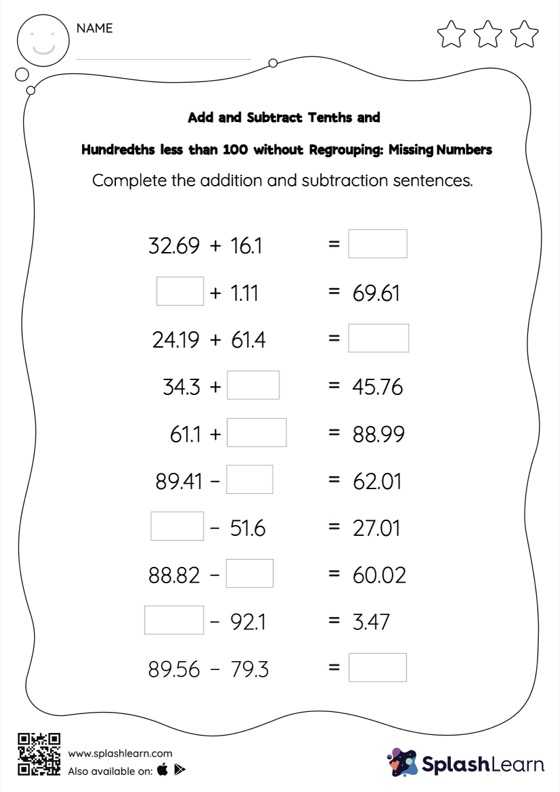# Add and Subtract Tenths and Hundredths less than 100 without Regrouping: Missing Numbers Worksheet

Home > Add and Subtract Tenths and Hundredths less than 100 without Regrouping: Missing NumbersAdd and subtract tenths and hundredths less than 100 without regrouping worksheet is a great way to help learners become fluent with the concept of addition and subtraction. Students use the relationship between addition and subtraction to find the missing number while adding and subtracting decimals. They do not need to regroup the numbers in add and subtract tenths and hundredths less than 100 without regrouping worksheet to get to the result. In each problem, the numbers are laid out in the horizontal format. Students should try to use different strategies involving composing and decomposing numbers to solve these problems. This will help them develop flexibility and fluency.